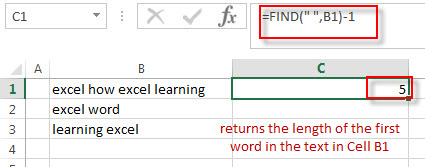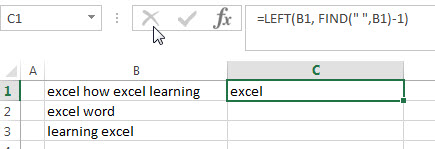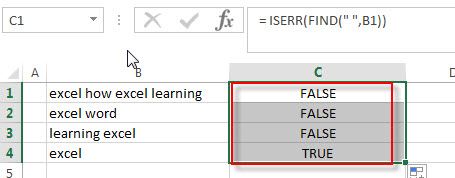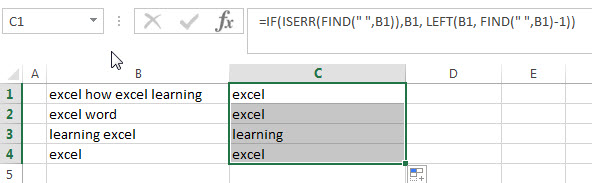# How to get first word from text string

This post will guide you how to extract the first word from a text string in excel. How to get the first word in a text using excel formula.

## Get First word

If you want to extract the first word from a text string in a cell, you can use a combination of the IF function, the ISERR function, the LEFT function and the FIND function to create a complex excel formula.

You can use the LEFT function to extract the first word, but the problem is that how to get the length of the first word in the text.  So you can use the FIND function to get the position of the first space character in the text in a cell, the number is then subtracted by 1 to get the length of the first word.

So Assuming that you want to get the first word in a text string in Cell B1, then you can write down an excel formula as follows:

`=IF(ISERR(FIND(" ",B1)),B1, LEFT(B1, FIND(" ",B1)-1))`

Let’s see how this formula works:

=FIND(” “,B1)-1This formula returns the length of the first word in the text in Cell B1. It goes into the LEFT function as its num_chars argument.

=LEFT(B1, FIND(” “,B1)-1)This formula extracts the first word in the text in cell B1. The LEFT function extracts the left-most characters and the number of characters that you need to extract is specified by the result returned by the FIND function.

= ISERR(FIND(” “,B1))The ISERR function will check if the FIND function do not find any space character in the text, it means that it contains only one word or a blank Cell. If

=IF(ISERR(FIND(” “,B1)),B1, LEFT(B1, FIND(” “,B1)-1))If the ISERR function returns TRUE, then returns the value of Cell B1, otherwise, returns the first word of the text in Cell B1.

### Related Formulas

• Split Multiple Lines from a Cell into Rows
If you have multiple lines in a cell and each line is separated by line break character or press “alt + enter” key while you entering the text string into cells, and you need to extract the multiple lines into the separated rows or columns, you can use a combination with the TRIM function, the MID function, the SUBSTITUTE function, the REPT function, the LEN function to create a complex excel formula..…
• Extract nth word from text string
If you want to extract the nth word from a text string in a single cell, you can create an excel formula based on the TRIM function, the MID function, the SUBSTITUTE function, the REPT function and the LEN function..…
• Get last word from text string
If you want to get the last word from a text string, you can create an excel formula based on the RIGHT function, the LEN function, the FIND function and the SUBSTITUTE function..…
• count specific words in a cell or a range
If you want to count the number of a specific word in a single cell, you need to use the SUBSTITUTE function to remove all that certain word in text string, then using LEN function to calculate the length of the substring that without that specific word.…
• Extract word that starting with a specific character
Assuming that you have a text string that contains email address in Cell B1, and if you want to extract word that begins with a specific character “@” sign, you can use a combination with the TRIM function, the LEFT function, the SUBSTITUTE function, the MID function, the FIND function, the LEN function and the REPT function to create an excel formula.…

### Related Functions

• Excel LEFT function
The Excel LEFT function returns a substring (a specified number of the characters) from a text string, starting from the leftmost character.The LEFT function is a build-in function in Microsoft Excel and it is categorized as a Text Function.The syntax of the LEFT function is as below:= LEFT(text,[num_chars])…t)…
• Excel IF function
The Excel IF function perform a logical test to return one value if the condition is TRUE and return another value if the condition is FALSE. The IF function is a build-in function in Microsoft Excel and it is categorized as a Logical Function.The syntax of the IF function is as below:= IF (condition, [true_value], [false_value])….
• Excel FIND function
The Excel FIND function returns the position of the first text string (sub string) within another text string.The syntax of the FIND function is as below:= FIND(find_text, within_text,[start_num])…
• Excel ISERR function
The Excel ISERR function returns TRUE if the value is any error value except #N/A.The syntax of the ISERR function is as below:= ISERR (value)…
Related Posts

Abbreviate Names Or Words in Excel

As an MS Excel user, you might have come across a task where you need to abbreviate different names or words, and there are also possibilities that you might have done this task manually by assuming that there isn't any ...

Filter with Multiple Criteria

If you are an valid MS Excel user, you have probably come across a situation where you wanted to filter the data in a separate table with complex criteria. You could do this task manually, which is also acceptable while ...

Extract substring In Excel

This post will guide you how to use Excel's MID function is a quick and easy way to extract pieces from your text. Use the Excel formula to extract a substring with MID. Note: If you want to extract just ...

How to Split Cells by the First Space in Texts in Excel

Sometimes we may use space to separate texts to different groups in one cell, if we want to split this cell to multiple columns refer to space, we can implement this via ‘Text to Columns’ feature. But is there any ...

How to Convert Uppercase to Lowercase Except the First Letter in Excel

In excel, words can be entered in uppercase, lowercase or mixed. If we want to convert all uppercase to lowercase (except the first letter in some situations), we can use formula with some letter convert related functions to convert them ...

Sidebar